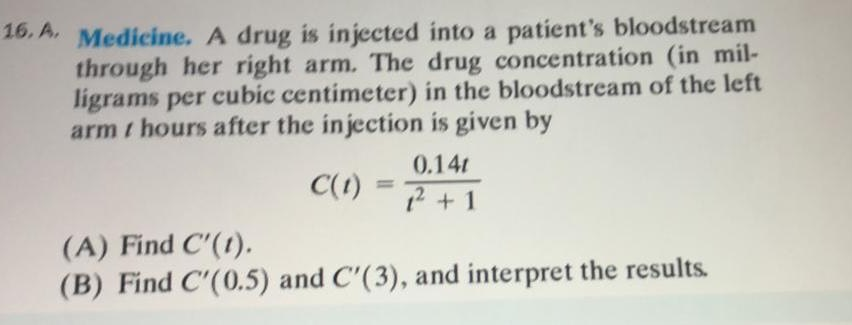### ¿Todavía tienes preguntas de matemáticas?

Pregunte a nuestros tutores expertos
Algebra
PreguntaA drug is injected into a patient's bloodstream through her right arm. The drug concentration (in milligrams per cubic centimeter) in the bloodstream of the left arm $$/$$ hours after the injection is given by

$$C ( t ) = \frac { 0.14 t } { t ^ { 2 } + 1 }$$

(A) Find $$C ^ { \prime } ( t )$$

(B) Find $$C ^ { \prime } ( 0.5 )$$ and $$C ^ { \prime } ( 3 ) ,$$ and interpret the results.

$$( \frac { 0.14 t } { t ^ { 2 } + 1 } ) ^ { \prime } = \frac { 0.14 ( - t ^ { 2 } + 1 ) } { ( t ^ { 2 } + 1 ) ^ { 2 } }$$